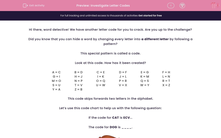# Investigate Letter Codes

In this worksheet, students will turn words into code and turn coded words back into their original words. This will develop student's encoding and problem-solving skills.This content is premium and exclusive to EdPlace subscribers.Key stage:  KS 2

Curriculum topic:   Verbal Reasoning

Curriculum subtopic:   Letter Code Breaking

Difficulty level:#### Worksheet Overview

Hi there, word detective! We have another letter code for you to crack. Are you up to the challenge?

Did you know that you can hide a word by changing every letter into a different letter by following a pattern?

This special pattern is called a code.

Look at this code. How has it been created?

 A = C B = D C = E D = F E = G F = H G = I H = J I = K J = L K = M L = N M = O N = P O = Q P = R Q = S R = T S = U T = V U = W V = X W = Y X = Z Y = A Z = B

This code skips forwards two letters in the alphabet.

Let’s use this code chart to help us with the following question:

If the code for CAT is ECV...

The code for DOG is ____.If we find D, then O, then G in the code chart, we can work out that the code FQI represents the word DOG.

Sometimes, we have to work backwards from a given code. Let’s look at this question as an example:

If RGC is the code for PEA, then VQR is the code for ____.

We need to start on the right-hand side and find the letters V, then Q, then R and record the associated letters.  If we do this, we find that the word is TOP.Pssst!!             Here's a handy hint to help you reach superstar status:             You may need to keep a pencil and paper handy to do your working out on. Use the code chart to work out the code for each word.

In this activity, you will need to be a code cracker and work out which code represents each word. Remember that if you are working backwards from a code, you will need to look at which letters changed to make them.

Good luck, code cracker!

### What is EdPlace?

We're your National Curriculum aligned online education content provider helping each child succeed in English, maths and science from year 1 to GCSE. With an EdPlace account you’ll be able to track and measure progress, helping each child achieve their best. We build confidence and attainment by personalising each child’s learning at a level that suits them.

Get started